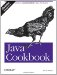# Recipe 16.5 Reading and Writing Binary Data

#### Problem

Having connected, you wish to transfer binary data.

#### Solution

Construct a DataInputStream or DataOutputStream from the socket's getInputStream( ) or getOutputStream( ).

#### Discussion

`DataInputStream is = new DataInputStream(sock.getInputStream( )); DataOutputStream is = new DataOutputStream(sock.getOutputStream( ));`

If the volume of data might be large, insert a buffered stream for efficiency. The paradigm is:

`DataInputStream is = new DataInputStream(     new BufferedInputStream(sock.getInputStream( ))); DataOutputStream is = new DataOutputStream(     new BufferedOutputStream(sock.getOutputStream( )));`

This program uses another standard service that gives out the time as a binary integer representing the number of seconds since 1900. Since the Java Date class base is 1970, we convert the time base by subtracting the difference between 1970 and 1900. When I used this exercise in a course, most of the students wanted to add this time difference, reasoning that 1970 is later. But if you think clearly, you'll see that there are fewer seconds between 1999 and 1970 than there are between 1999 and 1900, so subtraction gives the correct number of seconds. And since the Date constructor needs milliseconds, we multiply the number of seconds by 1,000.

The time difference is the number of years multiplied by 365, plus the number of leap days between the two dates (in the years 1904, 1908, . . . , 1968) i.e., 19 days.

The integer that we read from the server is a C-language unsigned int. But Java doesn't provide an unsigned integer type; normally when you need an unsigned number, you use the next-larger integer type, which would be long. But Java also doesn't give us a method to read an unsigned integer from a data stream. The DataInputStream method readInt( ) reads Java-style signed integers. There are readUnsignedByte( ) methods and readUnsignedShort( ) methods, but no readUnsignedInt( ) method. Accordingly, we synthesize the ability to read an unsigned int (which must be stored in a long , or else you'd lose the signed bit and be back where you started from) by reading unsigned bytes and reassembling them using Java's bit-shifting operators:

`\$ date Fri Mar 30 10:02:28 EST 2001 \$ java DaytimeBinary darian Remote time is 3194953367 BASE_DIFF is 2208988800 Time diff == 985964567 Time on darian is Fri Mar 30 10:02:47 EST 2001 \$`

Looking at the output, you can see that the server agrees within a few seconds. So the date calculation code in Example 16-6 is probably correct.

##### Example 16-6. DaytimeBinary.java
`/**  * DaytimeBinary - connect to the Time (binary) service.  */ public class DaytimeBinary {     /** The TCP port for the binary time service. */     public static final short TIME_PORT = 37;     /** Seconds between 1970, the time base for Date(long) and Time.      * Factors in leap years (up to 2100), hours, minutes, and seconds.      * Subtract 1 day for 1900, add in 1/2 day for 1969/1970.      */     protected static final long BASE_DAYS =          (long)((1970-1900)*365 + (1970-1900-1)/4)     /* Seconds since 1970 */     public static final long BASE_DIFF = (BASE_DAYS * 24 * 60 * 60);     /** Convert from seconds to milliseconds */     public static final int MSEC = 1000;     public static void main(String[] argv) {         String hostName;         if (argv.length == 0)             hostName = "localhost";         else             hostName = argv;         try {             Socket sock = new Socket(hostName, TIME_PORT);             DataInputStream is = new DataInputStream(new                  BufferedInputStream(sock.getInputStream( )));             // Need to read 4 bytes from the network, unsigned.             // Do it yourself; there is no readUnsignedInt( ).             // Long is 8 bytes on Java, but we are using the             // existing time protocol, which uses 4-byte ints.             long remoteTime = (                 ((long)(is.readUnsignedByte( ) ) << 24) |                 ((long)(is.readUnsignedByte( )) << 16) |                 ((long)(is.readUnsignedByte( )) <<  8) |                 ((long)(is.readUnsignedByte( )) <<  0));             System.out.println("Remote time is " + remoteTime);             System.out.println("BASE_DIFF is " + BASE_DIFF);             System.out.println("Time diff == " + (remoteTime - BASE_DIFF));             Date d = new Date((remoteTime - BASE_DIFF) * MSEC);             System.out.println("Time on " + hostName + " is " + d.toString( ));         } catch (IOException e) {             System.err.println(e);         }     } }`Java Cookbook, Second Edition
ISBN: 0596007019
EAN: 2147483647
Year: 2003
Pages: 409
Authors: Ian F Darwin

Similar book on Amazon